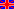http://www.wobsta.de/uni/tippetop/plots.shtml.en Tippe top: Numerical simulations plots »«Christian Friedl, André Wobst, 2005/05/25, 05:41 PMContentsModelFrictionElasticityMysteryExplanationPlotsAnimationInformations

# Numerical simulations – plots

The numerical solution of the equations of motion returns the real top behavior in a very pleasant way. We want to present a typical example with realistic top parameters: r = 1.5 cm, a = 0.3 cm, r' = 0.5 cm und a' = 1.9 cm.

One of the most interesting quantities is the inclination angle θ of the symmetry axis to the surface. The time dependent course shows the following figure:In the region of about 2 to 6 seconds the tippe top stands on its stem in our simulation. Afterwards one can recognise a plateau with fixed angle of 130 degree inclination which shows the so called double contact phase. In this state the stem and also the main sphere touches the surface and the respective bearing forces are determined by the elastic properties of top and surface.

At the beginning of the calculation the inclination angle is slightly shifted from zero, which is automatically satisfied in a real experiment. So we avoid that the top abides in the unstable equilibrium with exactly perpendicular symmetry axis. By the complicate interplay from whipping top and friction there arises a horizontal moving of the center of mass, which is projected on the x-y plane in the following picture:The different colors in this plot stands for different "phases" of the motion of the tippe top. The grey line signify, that only the main sphere touches the ground. The blue line shows the part of the motion where the top stands on its stem whereas the green line shows the case where both, the sphere and the stem, have ground contact. Finally there are a few short red segments where the top has taken off the surface! This happens mainly when the top rise up to the stem and when the main sphere falls back to the ground the first time.

Note, that we launched the top with a suitable initial velocity to keep the horizontal motion small. This is necessary to "hold the top in the picture" and we are able to create an animation of the gyration.

to the next section: Numerical simulations – animation

 Homepage  |  Theoretical physics I  |  Physics department  |  Augsburg University